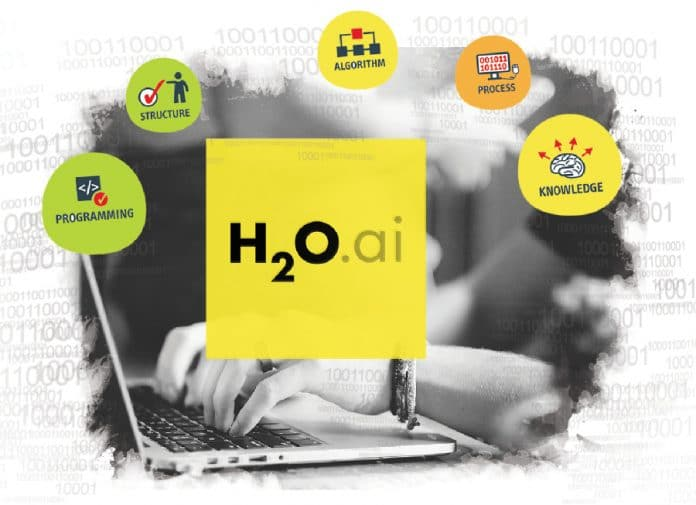# Introduction to H2O and its relation with deep learning

1
11053H2O, produced by the start up H2O.ai (formerly Oxdata), was launched in Silicon Valley in 2011. The speed of this open source software for big data analytics allows users to fit thousands of models for pattern discovery in data. H2O is written in Java, Python and R, and has many useful features on offer for deep learning.

Deep learning, defined simply, is ‘a class of machine learning techniques, where many layers of information processing stages in hierarchical supervised architectures are exploited for unsupervised feature learning and for pattern analysis/classification’. The essence of deep learning is to compute hierarchical features or representations of observational data, where higher-level features or factors are defined from lower-level ones. Although there are many similar definitions and architectures for deep learning, two common elements in all of them are: multiple layers of non-linear information-processing, and supervised or unsupervised learning of feature representations at each layer, from the features learned at the previous layer. The initial work on deep learning was based on multi-layer neural network models. Recently, many other models are also being used, such as deep kernel machines and deep Q-networks.

Introducing H2O
According to Oxdata (its creator), H2O is ‘the open source in-memory, prediction engine for Big Data science’. H2O is a feature-rich, open source machine learning platform known for its R and Spark integration and its ease of use. It is a Java virtual machine that is optimised for doing in-memory processing of distributed, parallel machine learning algorithms on clusters. A cluster is a software construct that can be fired up on your laptop, on a server, or across the multiple nodes of a cluster of real machines, including computers that form a Hadoop cluster.

Deep learning for IoT using H2O
In a nutshell, deep learning methods implement neural network algorithms such as the Feed Forward Back Propagation Neural Network, Convoluted Neural Networks, Recursive Neural Networks and others.
Deep learning algorithms play an important role in IoT analytics. Data from machines is sparse and/or has a temporal element in it. Even when we trust data from a specific device, it may behave differently under different conditions. Hence, capturing all scenarios for the data pre-processing/training stage of an algorithm is difficult. Monitoring sensor data continuously is also cumbersome and expensive. Deep learning algorithms can help to mitigate these issues. These algorithms learn on their own, allowing the developer to concentrate on more important tasks without worrying about training them.
Let’s now discuss what H2O offers as a part of its deep learning framework, and the features that make it suitable for data from things.

To boot H2O up in R from a local host, you should check the code. h20.init() provides the method to specify the IP, port and the number of threads to be used by H2O. By default, it uses all threads available in that machine. The first step is to start an instance of H2O. Using the Xmx parameter in the h2o.init() function, we can set aside the amount of RAM we want to use. I have 8GB of RAM on my machine; so I allocated 3GB to H2O.

```library(h2o)
localH2O = h2o.init(ip = “localhost”, port = 54321, startH2O = TRUE, Xmx = ‘3g’)
cat(“reading the training and testing data\n”)
train <- as.h2o(trainlocal)
test <- as.h2o(testlocal)```

Training a deep learning model
Once the data is loaded, the h20.deeplearning() method with appropriate parameters can be used to invoke the deep learning engine. The H2O deep learning package has various methods to pre-process the data itself. However, if we understand and know something about our data, we can very well use R’s native packages to pre-process it.
Shown below is sample code to invoke H2O’s deep learning package. The model I settled on has the following attributes:

• Rectified linear units as the activation function
• An input drop-out ratio of 0.2
• A hidden drop-out ratio of 0.5
• Neuron architecture of 784-800-800-10 (784 inputs, two hidden layers of 800 neurons each, and 10 Softmax output neurons)
• 500 epochs
``` s <- proc.time()
set.seed(1105)
model <- h2o.deeplearning(x = 2:785,
y = 1,
data = train,
activation = “RectifierWithDropout”,
input_dropout_ratio = 0.2,
hidden_dropout_ratios = c(0.5,0.5),
balance_classes = TRUE,
hidden = c(800,800),
epochs = 500)
e <- proc.time()
d <- e - s
d
model```

Here is a brief overview of the parameters used.

• X and Y: List of the predictors and target variables, respectively
• data: H2O training frame data
• activation: Indicates which activation function to use
• hidden: Number of hidden layers and their size
• l1: L1 regularisation
• train_samples_per_iteration: Number of training samples per iteration
• classification_stop: Stopping criterion for classification error
• epochs: How many times the dataset should be iterated
• balance_classes: If TRUE, balance the classes.

The model can be then applied to a new test dataset to validate its result using the h20.predict package.

```yhat <- h2o.predict(model, test)
ImageId <- as.numeric(seq(1,28000))
names(ImageId) <- “ImageId”
predictions <- cbind(as.data.frame(ImageId),as.data.frame(yhat[,1]))
names(predictions) <- “Label”
write.table(as.matrix(predictions), file=”training.csv”, row.names=FALSE, sep=”,”)```

Features that stand out for H2O
To summarise, the deep learning algorithm for IoT should have the following attributes:

• Be powerful enough to support distributed computing architectures to handle Big Data and do computations in parallel
• Be SMART enough
1.  Be able to pre-process and auto-impute the data itself without any external intervention
2.  Offer support for unsupervised feature learning
• Have the capability to prevent model overfitting
• Post process the data itself to give back results in the original form or unit of measure
• Cross-validate the results itself and decide if result optimisation is necessary

Almost all of these capabilities and features are demonstrated by H2O’s deep learning package. This makes it an ideal predictive analytics engine and a suitable choice to implement deep learning for the Internet of Things.#### 1 COMMENT

1.Mahshid ShIrani

Can we use different activation function for each layer in the network?